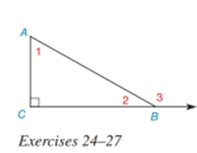Chapter 2.4, Problem 24E### Elementary Geometry for College St...

6th Edition
Daniel C. Alexander + 1 other
ISBN: 9781285195698

#### Solutions

Chapter
Section### Elementary Geometry for College St...

6th Edition
Daniel C. Alexander + 1 other
ISBN: 9781285195698
Textbook Problem
143 views

#Given: Right Δ A B C with right ∠ C m ∠ 1 = 7 x + 4 m ∠ 2 = 5 x + 2 Find: x

To determine

To find:

The value of x.

Explanation

Given:

m1=7x+4

And,

m2=5x+2

Figure (1)

Calculation:

Sum of acute angles in a right triangle is equal to 90°.

Equate the sum of acute angles in a right triangle to 90°.

m1+m2=90°(1)

Substitute 7x+4

### Still sussing out bartleby?

Check out a sample textbook solution.

See a sample solution

#### The Solution to Your Study Problems

Bartleby provides explanations to thousands of textbook problems written by our experts, many with advanced degrees!

Get Started

#### In Exercises 69-74, rationalize the numerator. 69. 2x3

Applied Calculus for the Managerial, Life, and Social Sciences: A Brief Approach

#### In Problems , find and.

Mathematical Applications for the Management, Life, and Social Sciences

#### For a given , the corresponding δ in the definition of is:

Study Guide for Stewart's Single Variable Calculus: Early Transcendentals, 8th

#### What is the primary factor that determines when it is time to change phases?

Research Methods for the Behavioral Sciences (MindTap Course List)